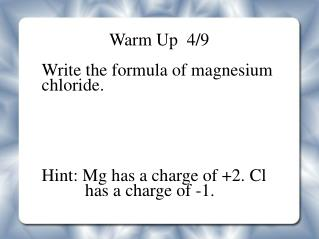# Warm Up 4/9 Write the formula of magnesium chloride. - PowerPoint PPT PresentationDownload PresentationWarm Up 4/9 Write the formula of magnesium chloride.

Warm Up 4/9 Write the formula of magnesium chloride.
Download Presentation## Warm Up 4/9 Write the formula of magnesium chloride.

- - - - - - - - - - - - - - - - - - - - - - - - - - - E N D - - - - - - - - - - - - - - - - - - - - - - - - - - -
##### Presentation Transcript

1. Warm Up 4/9 Write the formula of magnesium chloride. Hint: Mg has a charge of +2. Cl has a charge of -1.

2. Gas Laws

3. Lesson: Kinetic Molecular Theory

4. Solids/Liquids/Gases Describe the molecules in the following images.

5. Kinetic Molecular Theory A model that assumes that an ideal gas is composed of tiny particles (molecules) in constant motion. Ideal Gas- an imaginary gas that perfectly fits all of the KMT.

6. 5 Parts of KMT: 1. Matter is composed of very small particles called molecules. 2. Molecules are in constant motion. The collisions with the walls of the container cause pressure.

7. Continued 3. When the molecules collide with each other or the walls of a container, there is no loss of energy (elastic collision).

8. 4. There are no forces of attraction or repulsion between gas particles, but they collide and change direction.

9. 5. The average kinetic energy of gas particles depends on the temperature of the gas. Temperature- measure of the average kinetic energy of particles.

10. Pressure Units Standard atmosphere (atm) 1 atm= 760 mm Hg = 760 torr Pascal- SI unit for measuring pressure: 1 atm= 101300 Pa = 101.3 Kpa

11. Draw a picture for each of the parts of the KMT.

12. Warm Up If an atom has a charge of -2, has it been oxidized or reduced?

13. Lesson: Gas Laws (Part 1)

14. Gas Laws Gas laws express the behaviors of gases as mathematical relationships.

15. Boyle's Law -Pressure and volume -States that the pressure and volume of a gas are inversely proportional to each other at constant temperature.

16. Boyle's Law Formula: P1*V1 = P2*V2 P1= initial Pressure P2= final pressure V1= initial volume V2= final volume

17. Boyle’s Law Example‏ A gas has a volume of 1.25 L and a pressure of 755 torr. What will the new volume be if the pressure is increased to 2667.6 torr?

18. Charles' Law -Volume and temperature -The volume of a gas is directly proportional to the temperature at constant pressure.

19. Charles' Law Formula: V1= initial volume V2= final volume T1= initial temperature T2= final temperature **Temperature must be in Kelvin!

20. Charles’s Law Example 1‏ If a gas has a volume of 247 mL at 25.0°C, what will the new volume be if the temperature is increased to 308°C?

21. Charles’s Law Example 2‏ If a gas has a volume of 247 mL at 25.0°C, what will the new temperature be if the volume is increased to 255 mL?

22. Ideal Gas Law Formula: PV = nRT n = number of moles R = universal gas constant T = temperature (Kelvin) P= pressure (in atm) V = volume (in Liters)

23. Universal Gas Constant (R)‏ -value can vary depending on pressure units being used.

24. Example #1 (Ideal Gas Law)‏ What is the pressure of 5 moles of a gas at 30°C in a 2 liter container? P x 2 L = 5 mols x 0.0821 x 303 K 2 L 2 L P = 60 atm

25. Example #2 (Ideal Gas Law)‏ A 250 mL of a gas exerts a pressure of 760 torr at 10°C. How many moles of gas are present? 1 atm x 0.250 L = n x 0.0821 x 283 K (0.0821 x 283 K) 0.0821 x 283 K n = 0.0108 mol

26. 7) If I have 4 moles of a gas at a pressure of 5.6 atm and a volume of 12 liters, what is the temperature? 8) If I have an unknown quantity of a gas at a pressure of 1.2 atm, a volume of 31 liters and a temperature of 87°C, how many moles of gas do I have? 9) If I put 3 moles of gas in a container with a volume of 60 liters and at a temperature of 400 K, what is the pressure inside the container. 10) If I have 7.7 moles of a gas at a pressure of 0.09 atm and a temperature of 56°C, what is the volume of gas?

27. Get your notebooks! You will find your score near the last page.

28. What type of reaction? AgNO3 (aq) + KCl(aq) → AgCl (s) + KNO3 (aq)

29. What type of reaction? 2NaOH + H2SO4 → 2H2O + Na2SO4

30. What type of reaction? 2Mg + O2 → 2MgO

31. How many torr are in 2 atm?

32. What is the ideal gas formula?

33. Lesson: Gas Laws (Part 2)

34. Dalton's Law of Partial Pressures -states that the pressure of a gas mixture is the sum of the partial pressures of the individual components of the gas mixture. Formula: Ptotal = P1+P2+P3+...

35. Example (Partial Pressure)‏ A mixture of oxygen, hydrogen and nitrogen gases exerts a total pressure of 278 kPa. If the partial pressures of the oxygen and the hydrogen are 112 kPa and 101 kPa respectively, what would be the partial pressure exerted by the nitrogen?

36. Avogadro’s Law -Volume and number of moles -States that the volume and # of moles of a gas are directly proportional to each other at constant temperature and pressure.